Kiretu

# Reconstruction

To reconstruct a point cloud using Kinect, it is important to understand how reconstruction works in theory. Several steps are necessary but almost all of them base upon one fundamental formula, which will be derived in the following. It is taken from  and  (German).

Because it can be hard to understand the derivation for beginners, I tried to explain exery step in detail.

## Pinhole camera

We start with the model of a pinhole camera . Letbe a point in space which is mapped on the pointin the image plane.

To simplify the model, we mirror the image plane along the-axis in front of the camera between the optical center and the point:There are two coordinate-systems: The camera coordinate system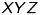and the image coordinate system. Note that the coordinates of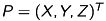andare arbitrary but fixed, so don’t mix them up with the coordinate systems.

We look at the scene above from the side: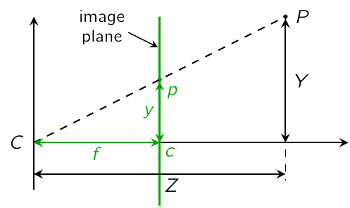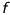is the distance between the optical center and the image plane and called the focal length. Due to the intercept theorem we get the following equations:The can combine these two equation to a vector:## Homogeneous coordinates

We now take a quick look at the most important characteristics of homogeneous coordinates. If you’ve never heard of homogeneous coordinates, you should catch up this topic.

1. Map a pointto its homogeneous coordinates:2. Equivalence of homogeneous coordinates:3. Get a pointgiven in homogeneous coordinates:You should keep these relations in mind.

We now map our points to their homogeneous coordinates as in (2):This leads us to our equation (1) in homogeneous coordinateswhere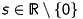represents the factorof (3). We can write this equation in the following way:## Principal point offset

We assumed that the origin of the image coordinate system is located in the images’s center, so far. But often that is not the case. Therefore, we add an offset to the image point:## Pixels at unit

Until now we usedand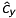in units of length, which is not appropriate to pixel-related digital images. Hence, we introduceand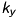, which are the number of pixels per unit of length ([pixel/length]) in- and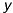-direction. We then get pixels as unit:with the camera matrix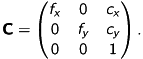## Transformation

As a last step, we have to consider the different position and orientation of the depth- and RGB-camera. To combine the two resulting coordinate systems, we use a transformation which includes a rotation and a transformation. This can be written as the following matrix: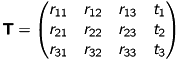We can now extend our equation (5) to:## Summary

The fundamental formuladescribes the relation between a threedimensional point in spacecaptured by a camera and its equivalent, twodimensional pointat the image plane.

The parameters are called:

•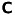: intrisic parameters or intrinsics
•: extrinsic parameters or extrinsics

It is important to understand, that our model (excepted the transformation) has been derived for one general camera. In context of Kinect you have seperate intrinsics for the depth- and RGB-camera!

In addation, we used the transformation to combine the coordinate systems of the depth- and RGB-camera. That implicates, that we only have got one transformation-matrix.

The application of our model/formula in context of Kinect is explained at class-description.

Hartley, Richard and Zisserman, Andres: Multiple View Geometry. Slides CVPR-Tutorial, 1999. http://users.cecs.anu.edu.au/~hartley/Papers/CVPR99-tutorial/tutorial.pdf
Kläser, Alexander: Kamerakalibrierung und Stereo Vision. Written report MIBI-Seminar, FH Bonn-Rhein-Sieg, 2005. http://www2.inf.fh-bonn-rhein-sieg.de/mi/lv/smibi/ss05/stud/klaeser/klaeser_ausarbeitung.pdf
Wikipedia (engl.): Pinhole camera model. http://en.wikipedia.org/wiki/Pinhole_camera_model [2012-01-22]

Date:
2012-01-26